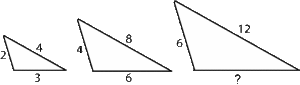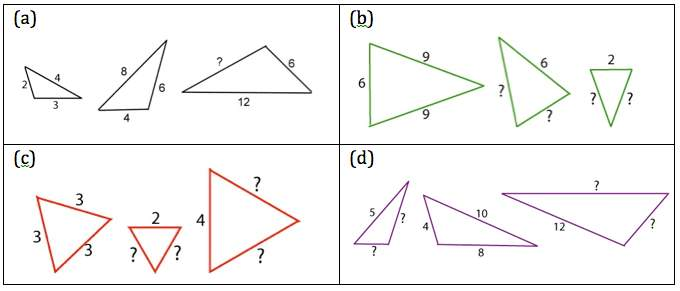Each picture shows 3 similar triangles.
They are called similar because they are the same shape, they have the same angles and the lengths of the edges are in the same ratio. For example in this diagram the edges in each triangle are all in the ratio 2 : 3 : 4 and (from left to right) the ratio of the edge lengths in the 3 triangles is 2 : 4 : 6 or equivalently 1 : 2 : 3. The triangles are enlargements of each other. The middle triangle is an enlargement of the triangle on the left by a scale factor 2, and the triangle on the right is an enlargement of the triangle on the left by a scale factor 3.

Find the missing lengths in the triangles below and explain how you found them.See AIMSSEC Teacher Notes SIMSETS

### One Response to Simsets

1.Mgcini Gwiji says:

Learners will have to recognise the corresponding sides from the two triangles. From there they look at the sizes of corresponding sides so that they can see the relationship in the given figures. In a way they have to check whether the sizes are increasing or decreasing, then find the pattern so that they can find missing side.